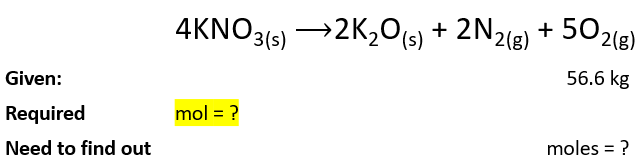# Problem: Potassium nitrate decomposes on heating, producing potassium oxide and gaseous nitrogen and oxygen: 4KNO3(s) ⟶2K2O(s) + 2N2(g) + 5O2(g) To produce 56.6 kg of oxygen, how many moles of KNO3 must be heated?

###### FREE Expert Solution

So, we have the mass of oxygen gas (product) in kg and we need to find out the number of moles of KNO3 (reactant) from it.

First, let’s have a look at the data.We will solve this problem through these steps:

• Convert mass of O2 from kg to g.
• Convert mass of O2 (in g) to mol.
• Convert moles of O2 to moles of KNO3 using a mole ratio in the above equation.

94% (339 ratings)###### Problem Details

Potassium nitrate decomposes on heating, producing potassium oxide and gaseous nitrogen and oxygen:

4KNO3(s) ⟶2K2O(s) + 2N2(g) + 5O2(g)

To produce 56.6 kg of oxygen, how many moles of KNO3 must be heated?